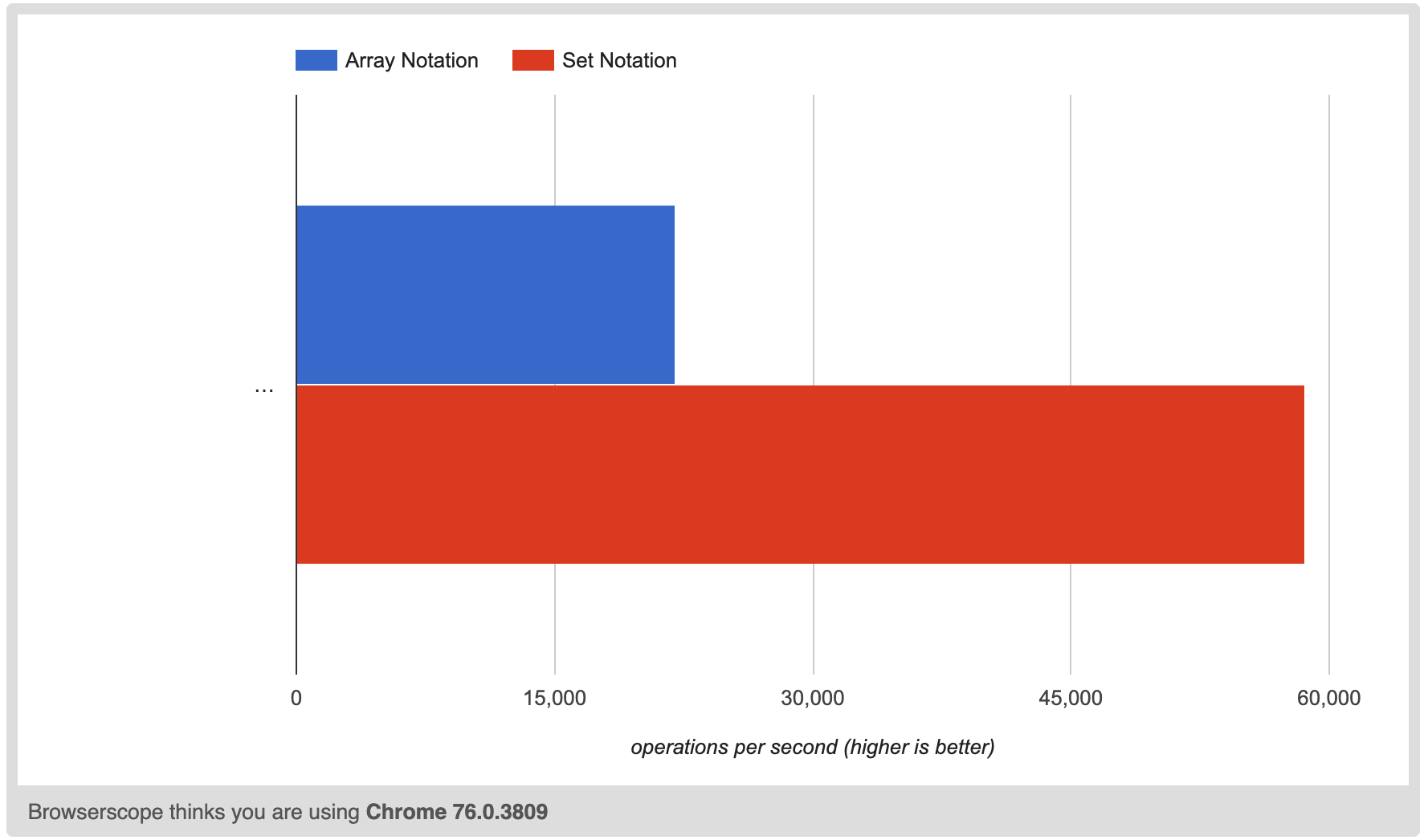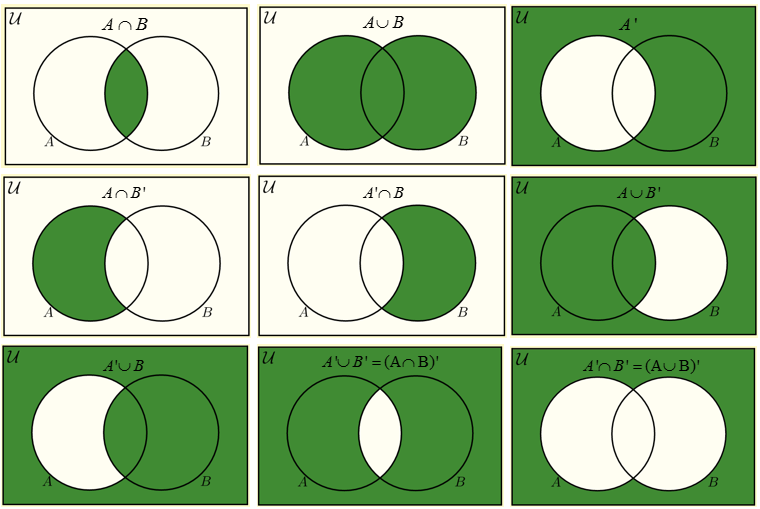# Use set to filter for uniqueness

October 08,'19 | Programming

Those who are have studied mathematics will be familiar with Sets. One working definition of a Set is "a collection of distinct things". This could be the list of numbers from one to ten, or it could be the types of fruit. But to many Javascript developers, the concept of a Set is novel. Why use Set at all? What are their use-cases?

For those who remember back to first-year algebra, this article seeks to remind you that Sets are a thing. To the remainder, this article sets to show that the Javascript Set fits a solid use-case, and that you can use it to solve your problem!

## What Exactly are Sets?

Sets are collections of unique things. When we denote sets in math, we usually use Capital letters to denote the sets, while using lower-case elements when we have to parameterize the things which are in the set. The following are sets:

A = { 'a', 'b', 'c', 'd', 'e', 'f' } B = { 1, 2, 3, 4, 5, 6 } C = { 🍊, 🥕, 🍎, 🍌 } D = The sequence of numbers from one to infinity E = { } F = The sequence of numbers of (3x^2), where x is itself a sequence of integers from 1 to 100

The following are not sets:

D = { 'a', 'a', 'b', 'c', 'd', 'e', 'f' } E = { 1, 2, 3, 4, 5, 6, 7, 4, 3, 1 } F = { 💀, 💀, 💀, 💀, 💀 } G = The sequence of numbers of f(x) = x^2 from x = -5 to x = 5, and x is an integer

These can't be sets because they show an element more than once. In Javascript-land, these are similar to arrays, or collections of things.

# Creating a New Set

The following is the syntax for creating a new set in Javascript:

`const arr = [1,2,3,4,5]const arrSet = new Set(arr)arrSet // -> Set {1,2,3,4,5}`

What happens is that when a set is declared, it takes a series of arguments. For all the arguments which are not unique, it only adds them once. For example:

`const arr = [9, 4, 1, 0, 1, 4, 9]const arrSet = new Set(arr)arrSet // -> Set { 9, 4, 1, 0 } `

As one can guess, this can become quickly useful if we want to have a collection of all the possible things, as opposed to just a collection of all the things. This quickly becomes useful if you want to carry out filtration operations for uniqueness. This is excellent for comparing, and is much faster than our familiar filter function below:

const filteredArr = arr.filter((i, index) => arr.indexOf(i) === index)

Here is a simple Set vs Array.filter() benchmark. Here you can see that using a set to filter for unique values is almost three times faster than using plain filters.It's one thing to keep in the realm of one-dimensional unique things. But as we know, I carry out a lot of my work in at least two dimensions given the applied realm of geography. For coordinates, we cannot compare arrays of coordinates in Javascript like we would in Python or Ruby. Here, we have to reach out for creative operations. Below combines the use of Set with the Javascript Map datatype:

`const uniqueCoordinates = coordinates => {  const map = new Map();  return coordinates.filter(coordinate => {    const [y, x] = coordinate;    if (!map[y]) map[y] = new Set();    const set = map[y];    if (set.has(x)) return false;    set.add(x);    return true;  });};`

This is not as fast as other filtering applications of Set, but it is still a boost over classic filtration. But for Javascript sets, filtering is not the only decent use-case. It turns out that there are a wide other array of these things, as well!

## Carrying out mathematical operations.

If you haven't already been to the mozilla docs (https://developer.mozilla.org/en-US/docs/Web/JavaScript/Reference/Global_Objects/Set#Implementing_basic_set_operations), there is some decent extensions of the Set operator that will help you on your way towards remembering all those things you forgot in first year linear algebra. You can compare two sets directly, or carry out Set algebra as well! The following are some examples which make use of the docs above.

### Subsets and Supersets

So imagine you have two sets, A and B. Some elements in A are within B, but not all elements in B are in A. One such example of this scenario is as follows:

`const A = new Set([1,2,3,4,5])     // -> Set{1,2,3,4,5}const B = new Set([1,2,3,4,5,6,7]) // -> Set{1,2,3,4,5,6,7}`

In this example,

• Set A is a subset of B (or A ⊆ B)

• Set B is a Superset of A (or B ⊇ A)

To calculate this, you will need to build your own function, which I took from MDN:

`const isSuperset = ({set, subset}) => {  for (let elem of subset) {    if (!set.has(elem)) {      return false;    }  }  return true;}isSuperset({set: B, subset: A}) // trueisSuperset({set: A, subset: B}) // false`

It's worth noting that if A isn't a subset of B, then B can't be a superset of A.

## Unions, and Intersections

As developers, we should remember those venn diagrams. Below is a chart of all the union and intersection operators.By looking at this chart, you can tell the following:

1. A UNION B is all the things in set A, plus all the things in set B as its own set of unique items.

2. A INTERSECT B is all the things that are common to sets A and B.

Set notion is fantastic for these things as well!

## But how useful is it, really?

Okay, if you're looking at this site, you know that I deal in maps. Coordinate pairs. Operations on coordinate pairs.

Well, it turns out that you can't use set notation on Objects or Arrays for the sake of uniqueness...

`const C = new Set([{lat: 123, lng: 123}, {lat: 123, lng: 123}, {lat: 0, lng: 0}])console.log(C)// -> Set {//  { lat: 123, lng: 123 },//  { lat: 123, lng: 123 },//  { lat: 0, lng: 0 } }const D = new Set([[0,0], [0,0], [0,1]])console.log(D)// -> Set { [ 0, 0 ], [ 0, 0 ], [ 0, 1 ] }`

As you can see, for geographic operations, the Set isn't the most useful right away. But that doesn't discount other use cases.